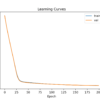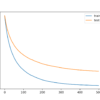# Author Archive | Jason Brownlee## How to Implement Gradient Descent Optimization from Scratch

Gradient descent is an optimization algorithm that follows the negative gradient of an objective function in order to locate the minimum of the function. It is a simple and effective technique that can be implemented with just a few lines of code. It also provides the basis for many extensions and modifications that can result […]## What Is a Gradient in Machine Learning?

Gradient is a commonly used term in optimization and machine learning. For example, deep learning neural networks are fit using stochastic gradient descent, and many standard optimization algorithms used to fit machine learning algorithms use gradient information. In order to understand what a gradient is, you need to understand what a derivative is from the […]Gradient descent is an optimization algorithm that follows the negative gradient of an objective function in order to locate the minimum of the function. A limitation of gradient descent is that it uses the same step size (learning rate) for each input variable. AdaGradn and RMSProp are extensions to gradient descent that add a self-adaptive […]## What Is Semi-Supervised Learning

Semi-supervised learning is a learning problem that involves a small number of labeled examples and a large number of unlabeled examples. Learning problems of this type are challenging as neither supervised nor unsupervised learning algorithms are able to make effective use of the mixtures of labeled and untellable data. As such, specialized semis-supervised learning algorithms […]## Develop a Neural Network for Cancer Survival Dataset

It can be challenging to develop a neural network predictive model for a new dataset. One approach is to first inspect the dataset and develop ideas for what models might work, then explore the learning dynamics of simple models on the dataset, then finally develop and tune a model for the dataset with a robust […]## Neural Network Models for Combined Classification and Regression

Some prediction problems require predicting both numeric values and a class label for the same input. A simple approach is to develop both regression and classification predictive models on the same data and use the models sequentially. An alternative and often more effective approach is to develop a single neural network model that can predict […]## Iterated Local Search From Scratch in Python

Iterated Local Search is a stochastic global optimization algorithm. It involves the repeated application of a local search algorithm to modified versions of a good solution found previously. In this way, it is like a clever version of the stochastic hill climbing with random restarts algorithm. The intuition behind the algorithm is that random restarts […]## Develop a Neural Network for Woods Mammography Dataset

It can be challenging to develop a neural network predictive model for a new dataset. One approach is to first inspect the dataset and develop ideas for what models might work, then explore the learning dynamics of simple models on the dataset, then finally develop and tune a model for the dataset with a robust […]## Tune XGBoost Performance With Learning Curves

XGBoost is a powerful and effective implementation of the gradient boosting ensemble algorithm. It can be challenging to configure the hyperparameters of XGBoost models, which often leads to using large grid search experiments that are both time consuming and computationally expensive. An alternate approach to configuring XGBoost models is to evaluate the performance of the […]## Two-Dimensional (2D) Test Functions for Function Optimization

Function optimization is a field of study that seeks an input to a function that results in the maximum or minimum output of the function. There are a large number of optimization algorithms and it is important to study and develop intuitions for optimization algorithms on simple and easy-to-visualize test functions. Two-dimensional functions take two […]